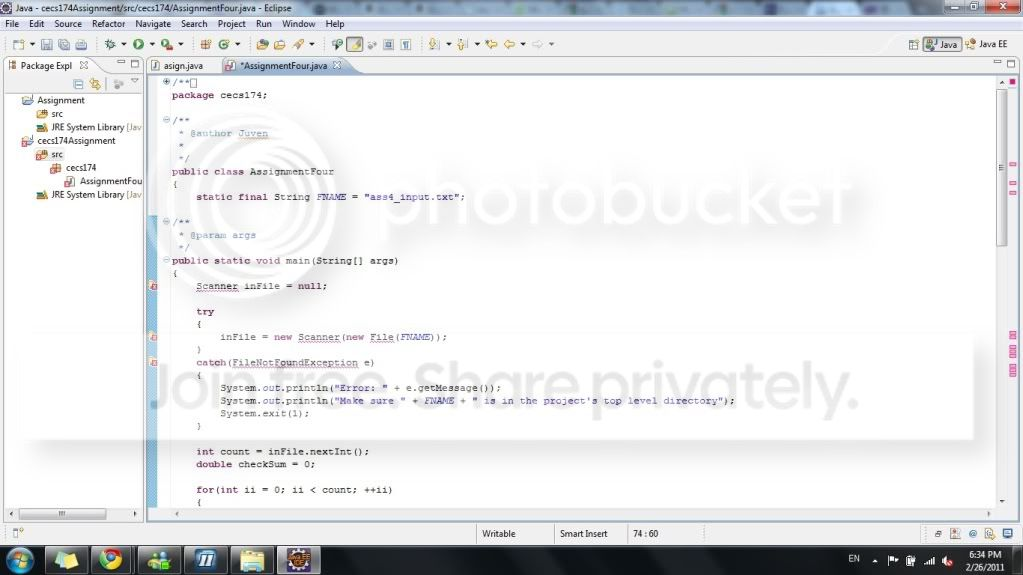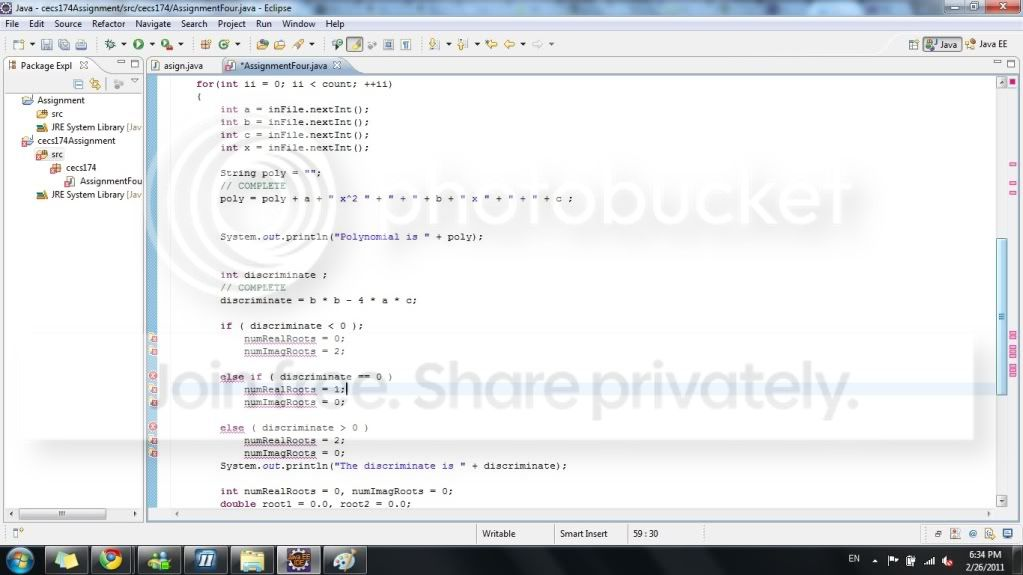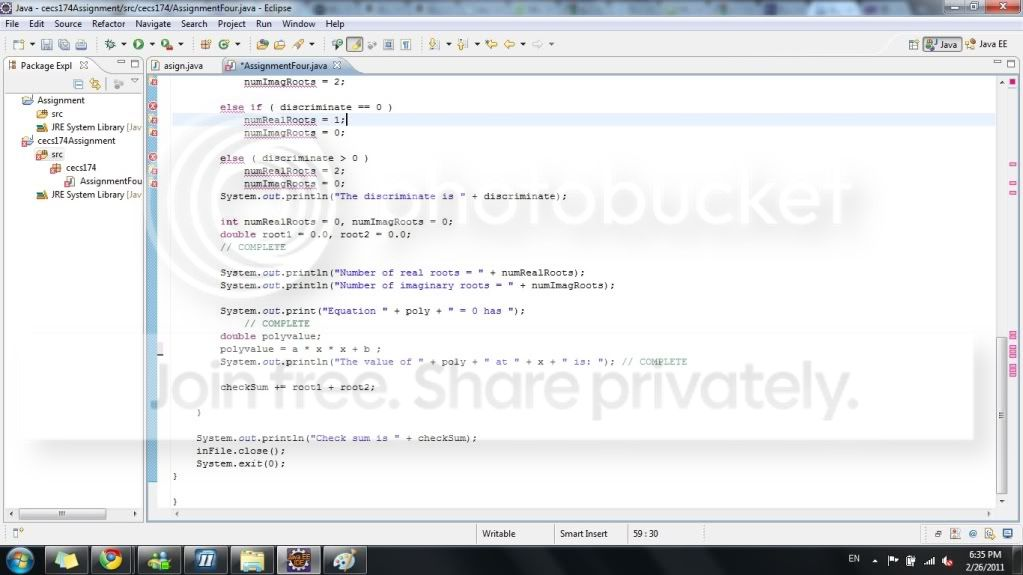# Thread: Need Help For doing if else statement

1.MemberJoin Date
Feb 2011
Posts
7
Rep Power
0

##Need Help For doing if else statement

First of all, I never learn any java or C programming.. so I am new to any programming.

I got an assignment with skeleton provided from professor, but there are errors and I do not know how to fix it.
It is really easy to you guys.. right?
I want to finish this assignment so that I can focus on my other classes..
i will also post what I wrote in #2:D
Thanks for helping me...:o
He gave us simple instruction, input and desire output which are the following:

From Prof. :This assignment will give you practice with if statements, strings, polynomials of degree 2 and Math.sqrt.

The file available on the Web page is the main(). I have written some of it and you must complete it. The sections to be
completed are marked // Complete.

I will run your program using the input file posted on the Web page. Your output must match the desired output posted on the Web page. Be sure to test the following values:

a < -1, a == -1, a = 1, a > 1, b < -1, b == -1, b == 0, b == 1, b > 1
c < -1, c == -1, c == 0, c == 1, c > 1.

Input:
Java Code:
```8
-2	-2	-2	1
-1	-1	-1	2
1	-1	-1	1
1	 0	 0     -1
1	 1	 1     -2
2	 2	 2	1
1	 0	-4 	0
1 	 0     -25 	1```

Skeleton:
Java Code:
```/**
*
*/
package cecs174;

import java.io.File;
import java.util.Scanner;
import java.io.FileNotFoundException;

/**
* @author murgolo
*
*/
public class AssignmentFourMain
{
static final String FNAME = "ass4_input.txt";
/**
* @param args
*/
public static void main(String[] args)
{
Scanner inFile = null;

try
{
inFile = new Scanner(new File(FNAME));
}
catch(FileNotFoundException e)
{
System.out.println("Error: " + e.getMessage());
System.out.println("Make sure " + FNAME + " is in the project's top level directory");
System.exit(1);
}

int count = inFile.nextInt();
double checkSum = 0;

for(int ii = 0; ii < count; ++ii)
{
int a = inFile.nextInt();
int b = inFile.nextInt();
int c = inFile.nextInt();
int x = inFile.nextInt();

String poly = "";
// COMPLETE

System.out.println("Polynomial is " + poly);

int discriminate ;
// COMPLETE

System.out.println("The discriminate is " + discriminate);

int numRealRoots = 0, numImagRoots = 0;
double root1 = 0.0, root2 = 0.0;
// COMPLETE

System.out.println("Number of real roots = " + numRealRoots);
System.out.println("Number of imaginary roots = " + numImagRoots);

System.out.print("Equation " + poly + " = 0 has ");
// COMPLETE

System.out.println("The value of " + poly + " at " + x + " is: "); // COMPLETE

checkSum += root1 + root2;

}

System.out.println("Check sum is " + checkSum);
inFile.close();
System.exit(0);
}

}```

Desire output

(a,b,c,x) = (-2,-2,-2,1)
Polynomial is -2x^2 - 2x - 2
The discriminate is -12
Number of real roots = 0
Number of imaginary roots = 2
Equation -2x^2 - 2x - 2 = 0 has no real roots
The value of -2x^2 - 2x - 2 at 1 is: -6

(a,b,c,x) = (-1,-1,-1,2)
Polynomial is -x^2 - x - 1
The discriminate is -3
Number of real roots = 0
Number of imaginary roots = 2
Equation -x^2 - x - 1 = 0 has no real roots
The value of -x^2 - x - 1 at 2 is: -7

(a,b,c,x) = (1,-1,-1,1)
Polynomial is x^2 - x - 1
The discriminate is 5
Number of real roots = 2
Number of imaginary roots = 0
Equation x^2 - x - 1 = 0 has two real roots = (1.618033988749895,-0.6180339887498949)
The value of x^2 - x - 1 at 1 is: -1

(a,b,c,x) = (1,0,0,-1)
Polynomial is x^2
The discriminate is 0
Number of real roots = 1
Number of imaginary roots = 0
Equation x^2 = 0 has one real root = 0.0
The value of x^2 at -1 is: 1

(a,b,c,x) = (1,1,1,-2)
Polynomial is x^2 + x + 1
The discriminate is -3
Number of real roots = 0
Number of imaginary roots = 2
Equation x^2 + x + 1 = 0 has no real roots
The value of x^2 + x + 1 at -2 is: 3

(a,b,c,x) = (2,2,2,1)
Polynomial is 2x^2 + 2x + 2
The discriminate is -12
Number of real roots = 0
Number of imaginary roots = 2
Equation 2x^2 + 2x + 2 = 0 has no real roots
The value of 2x^2 + 2x + 2 at 1 is: 6

(a,b,c,x) = (1,0,-4,0)
Polynomial is x^2 - 4
The discriminate is 16
Number of real roots = 2
Number of imaginary roots = 0
Equation x^2 - 4 = 0 has two real roots = (2.0,-2.0)
The value of x^2 - 4 at 0 is: -4

(a,b,c,x) = (1,0,-25,1)
Polynomial is x^2 - 25
The discriminate is 100
Number of real roots = 2
Number of imaginary roots = 0
Equation x^2 - 25 = 0 has two real roots = (5.0,-5.0)
The value of x^2 - 25 at 1 is: -24
Check sum is 1.0
Last edited by Fubarable; 02-27-2011 at 02:23 AM.Reply With Quote

2. ##Moderator edits:

1) moved from Eclipse forum to the New To Java forum for closer forum-topic alignment.Reply With Quote

3.MemberJoin Date
Feb 2011
Posts
7
Rep Power
0

##This is how far I go..
I don't know how to write .... I am really stuck at this point.:(
The following is the things I wrote.. and a printscreen (to let u see the errors):)

/**
*
*/
package cecs174;

/**
* @author Juven
*
*/
public class AssignmentFour
{
static final String FNAME = "ass4_input.txt";

/**
* @param args
*/
public static void main(String[] args)
{
Scanner inFile = null;

try
{
inFile = new Scanner(new File(FNAME));
}
catch(FileNotFoundException e)
{
System.out.println("Error: " + e.getMessage());
System.out.println("Make sure " + FNAME + " is in the project's top level directory");
System.exit(1);
}

int count = inFile.nextInt();
double checkSum = 0;

for(int ii = 0; ii < count; ++ii)
{
int a = inFile.nextInt();
int b = inFile.nextInt();
int c = inFile.nextInt();
int x = inFile.nextInt();

String poly = "";
// COMPLETE
poly = poly + a + " x^2 " + " + " + b + " x " + " + " + c ;

System.out.println("Polynomial is " + poly);

int discriminate ;
// COMPLETE
discriminate = b * b - 4 * a * c;

if ( discriminate < 0 );
numRealRoots = 0;
numImagRoots = 2;

else if ( discriminate == 0 )
numRealRoots = 1;
numImagRoots = 0;

else ( discriminate > 0 )
numRealRoots = 2;
numImagRoots = 0;
System.out.println("The discriminate is " + discriminate);

int numRealRoots = 0, numImagRoots = 0;
double root1 = 0.0, root2 = 0.0;
// COMPLETE

System.out.println("Number of real roots = " + numRealRoots);
System.out.println("Number of imaginary roots = " + numImagRoots);

System.out.print("Equation " + poly + " = 0 has ");
// COMPLETE
double polyvalue;
polyvalue = a * x * x + b * x + c ;

System.out.println("The value of " + poly + " at " + x + " is: "); // COMPLETE

checkSum += root1 + root2;

}

System.out.println("Check sum is " + check Sum);
inFile.close();
System.exit(0);
}

}Reply With Quote

4.ModeratorJoin Date
Feb 2009
Location
New Zealand
Posts
4,716
Rep Power
18

##The usual way of doing things is to post the compiler messages if you can't understand them.

In this case one source of error stands out straight away: use braces with every if and else block, even ones that are only one line long.

Java Code:
```if(something) [b][color=red]{[/color][/b]
// code
[b][color=red]}[/color][/b] else if(somethingElse) [b][color=red]{[/color][/b]
// code
[b][color=red]}[/color][/b] else [b][color=red]{[/color][/b]
// code
[b][color=red]}[/color][/b]```Reply With Quote

5.MemberJoin Date
Feb 2011
Posts
7
Rep Power
0

##oh.. braces for every if .. else if..
where should i put my ass4_input.txt ??
there are three errors around the scanner file.. how to I fix that.

Thanks pbrockwayReply With Quote

6.MemberJoin Date
Feb 2011
Posts
7
Rep Power
0

##comparing the desire out put and my code..
am I right to put numRealRoots and numImagRoots in that place?Reply With Quote

7.ModeratorJoin Date
Feb 2009
Location
New Zealand
Posts
4,716
Rep Power
18

##Originally Posted by juvenchanoh.. braces for every if .. else if..
where should i put my ass4_input.txt ??
there are three errors around the scanner file.. how to I fix that.

Thanks pbrockway
You're welcome.

Please post the compiler messages. I *think* you have not imported the Scanner class or the FileNotFoundException class, so the compiler will complain about that.

One thing: when you post code use the "code" tags. Put [code] at the start of the code and [/code] at the end. That will preserve the formatting. Most people (including me!) are more inclined to read the code if it is properly formatted.Reply With Quote

8.MemberJoin Date
Feb 2011
Posts
7
Rep Power
0

##Originally Posted by pbrockway2You're welcome.

Please post the compiler messages. I *think* you have not imported the Scanner class or the FileNotFoundException class, so the compiler will complain about that.

One thing: when you post code use the "code" tags. Put [code] at the start of the code and [/code] at the end. That will preserve the formatting. Most people (including me!) are more inclined to read the code if it is properly formatted.
Exception in thread "main" java.lang.Error: Unresolved compilation problems:
Scanner cannot be resolved to a type
Scanner cannot be resolved to a type
File cannot be resolved to a type
FileNotFoundException cannot be resolved to a type
numRealRoots cannot be resolved to a variable
numImagRoots cannot be resolved to a variable
numRealRoots cannot be resolved to a variable
numImagRoots cannot be resolved to a variable
Syntax error on token ")", } expected
numRealRoots cannot be resolved to a variable
numImagRoots cannot be resolved to a variable
Syntax error on token "}", delete this token
Syntax error, insert "}" to complete ClassBody

at cecs174.AssignmentFour.main(AssignmentFour.java:19 )

I don't get what u mean by using
Java Code:
`put my codes here?`
sorry that I am a newbie in java or any programmingReply With Quote

9.ModeratorJoin Date
Feb 2009
Location
New Zealand
Posts
4,716
Rep Power
18

##Did you understand what I meant by having to include Scanner and FileNotFoundException?

Find out what package they belong to and put an import line for each just below the package statement at the top. (Check notes/textbook etc for examples of this.)

numRealRoots cannot be resolved to a variable

This means that you have not declared numRealRoots anywhere (you have to tell the compiler that it's an int). You need a line like

Java Code:
`int numRealRoots;`

before you use that variable. You did that for discriminant and you have to do it for all variables.Reply With Quote

10.MemberJoin Date
Feb 2011
Posts
7
Rep Power
0

##after u see the desire output.
how should I declare the numRealRoots and numImagRoots?
do u mind give me a hand, or .. help me to write it?
i don;t have the java book with me..
oh boy.. java really makes me headache.. haha
r u cs major?Reply With Quote

11.ModeratorJoin Date
Feb 2009
Location
New Zealand
Posts
4,716
Rep Power
18

##I don't write code for people.

As far as including the necessary imports and declaring variables before use is concerned (and that's a fair number of the compiler messages) your best bet is to consult some text or online tutorial. Oracle's Tutorial covers the basics in the section Learning the Java Language.

Many other online tutorials exist - including one linked to here within the lat couple of days that looked very good.Reply With Quote

12.MemberJoin Date
Feb 2011
Posts
7
Rep Power
0

##oh..
i think i wrong it in the wrong place..
i replace it.. it shows no error..for that part..
but the scanner still cannot be resolved...... interesting....Reply With Quote

####Posting Permissions

• You may not post new threads
• You may not post replies
• You may not post attachments
• You may not edit your posts
•